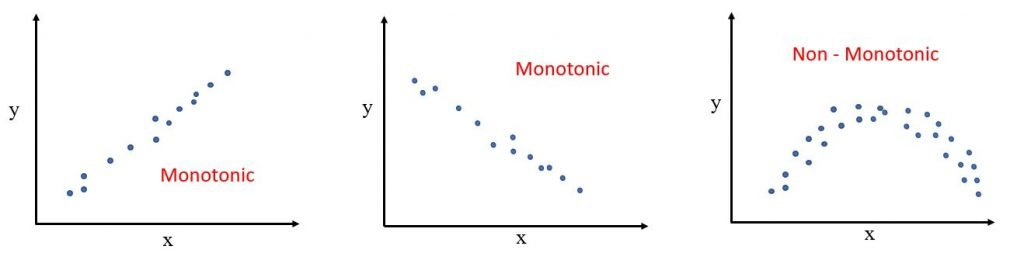# Correlation Coefficient Interpretation## Introduction

The correlation coefficient is used to measure the strength of the relationship between two variables. There are several types of correlation coefficients;

1. Pearson Correlation Coefficient
2. Spearman Rank Correlation Coefficient
3. Kendall Rank Correlation Coefficient

## Pearson Correlation Coefficient

Generally, when we say correlation coefficient, we actually mean Pearson’s Correlation Coefficient.

This is used to measure the strength of the relationship between two continuous variables.

It’s value is between -1 to +1.

A value closer to +1 means there is a strong positive relationship between the two variables. Which means, when one variable increases, the other also increases.

Example : Marks of students tend to increase when their attendance increase.

A value closer to -1 means there is a strong negative relationship between the two variables. This means, when one variable increases, the other one also decreases.

Example : Marks of students decrease when they watch more television.

Pearson correlation coefficient can be called as the best method of measuring the relationship between two variables because it is based on the method of covariance.

\begin{equation} \rho_{x,y} = \dfrac{Cov(X,Y) }{\sigma_{X}\sigma_{Y}} \end{equation}

## Spearman Rank Correlation Coefficient

This is a non parametric test used to measure the relationship between two variables. It is ideal when data is in ordinal scale.

Spearman Rank Correlation Coefficient is not based on any assumptions about the distribution of data.

Example : Relationship between a person’s level of education and monthly income

But, it assumed that data is at least of ordinal scale and scores on one variable is monotonically related to the other variable.

### What is a monotonic relationship?

A monotonic relationship is a relationship where when one variable increases, the other one also increases or when one decreases, the other one increases.

## Kendall Rank Correlation Coefficient

Kendall Rank Correlation Coefficient is a non-parametric test used to measure relationship between two variables. Since it is a non parametric test, it does not depend on the distribution of the underlying data.

It is based on the ranks of data. It’s value is either 0 or 1

0 means no relationship and 1 means a perfect relationship.

Sometimes, this test procedure produces negative values. Since we deal with ranked data, there is no meaning to a negative value. Therefore, if you come across a negative sign, just ignore it during interpretations.